Mandić, M., Porankiewicz, B., and Danon, G. (2015). "An attempt at modelling of cutting forces in oak peripheral milling," BioRes. 10(3), 5489-5502.

#### Abstract

An attempt was made to evaluate the non-linear, multi-variable dependency between the main (tangential) force, FC, and machining parameters and properties of pedunculate oak (Quercus robur) during straight edge, peripheral milling. The tangential force, FC, was found to be influenced by the feed rate per tooth, fZ, cutting depth, cD, rake angle γF, Brinell hardness, H, bending strength, RB, and modulus of longitudinal elasticity, E. Several interactions between machining parameters and properties of wood were confirmed in the developed relationship FC = f(fZ, cD, γF, H, RB, E).

#### Full Article

An Attempt at Modelling of Cutting Forces in Oak Peripheral Milling

Marija Mandić,a Bolesław Porankiewicz,b,* and Gradimir Danon a

An attempt was made to evaluate the non-linear, multi-variable dependency between the main (tangential) force, FC, and machining parameters and properties of pedunculate oak (Quercus robur) during straight edge, peripheral milling. The tangential force, FC, was found to be influenced by the feed rate per tooth, fZ, cutting depth, cD, rake angle γF, Brinell hardness, H, bending strength, RB, and modulus of longitudinal elasticity, E. Several interactions between machining parameters and properties of wood were confirmed in the developed relationship FC = f(fZ, cD, γF, H, RB, E).

Keywords: Oak; Properties of wood; Machining parameters; Peripheral milling; Main cutting force

Contact information: a: Department of Wood Processing, University of Belgrade- Faculty of Forestry, Belgrade, Serbia; b: Poznań University of Technology, Poland;

* Corresponding author: poranek@amu.edu.pl

INTRODUCTION

It seems that contemporary theory of wood machining was developed on the basis of metal processing theory (Afanasev 1961; Beršadskij 1967; Glebov 2007; Manžos 1974; Amalitskij and Lûbčenko 1977). An attempt to base wood cutting theory on the mechanical properties of wood for three main and intermediate cutting directions (modes) has already been done (Deševoy 1939). Factors that impact the mechanics of wood cutting (and chip formation) can be divided into three groups (Eyma et al. 2004; Naylor and Hackney 2013): wood species, wood properties, and the cutting process itself. In the work of Franz (1958) aimed at main and normal cutting force analysis (with the use of a dynamometer equipped with an electrical resistance strain-gage bridge and strain-analyzing instrument), several mechanical properties (tensile strength perpendicular to grain, RT⊥, modulus of elasticity in tensile parallel to grain, E, modulus of rupture in bending, RB, modulus of elasticity in bending, EB, crushing strength parallel to grain, RC॥, and shear strength parallel to grain, RS॥, and cleavage, RCL) were evaluated for moisture contents of 3%, 11%, and 30%, and for wood specimens of yellow birch (Betula alleghaniensis Britt.), sugar pine (Pinus lambertiana Dougl.), and white ash (Fraxinus americana L.).

The papers of various researchers (Kivimaa 1950; Franz 1958; McKenzie 1960; Koch 1964; Woodson and Koch 1970; Axelsson et al. 1993; McKenzie et al. 1999) have discussed the impacts of the stated factors on cutting forces and chip formation, durability of tools, precision of processing, and quality of the processed surface. The primary objective of these studies was to better understand the interaction between a tool and processed item for the purpose of more efficient management of cutting processes. Energy and tool saving methods are also important. However, in this case, they are in the background.

In the literature dealing with mechanical wood processing, various methods for anticipating/calculating cutting forces can be found. In many approaches, these are coefficient methods, where authors start from a referent unit cutting resistance for a certain wood species, most frequently pine wood, which is calculated under strictly defined and controlled (standard) conditions (Afanasev 1961; Beršadskij 1967; Manžos 1974; Amalitskij and Lûbčenko 1977; Orlicz 1982; Goglia 1994; Glebov 2007). Specific cutting resistances for certain materials and certain cutting conditions (for all possible wood grains orientation angles or cutting modes) are obtained by multiplying referent unit cutting resistances and appropriate correction coefficients, which can be found in adequate tables. Orlicz (1982) pointed out that differences between forces calculated using the coefficient method and forces measured in experiments reached as high as 40%. In certain cases, this difference was more than 100% (Mandić et al. 2014). There are several possible reasons for the discrepancies, but one of the primary reasons is the fact that physical and mechanical properties of wood are not sufficiently and properly included in the stated models. It is known from the literature that physical and mechanical properties of wood depends on the environmental conditions in which the tree grew up, as well as on the position of the longitudinal and transverse cross-section of the trunk (Krzysik 1975).

The work of Eyma et al. (2004) would be an interesting, novel approach of employing several mechanical properties of wood of 13 species to cutting forces analysis. However, instead of main (tangential) cutting force, FC, the resultant cutting force, FR=(FC2+FN2)0.5 was taken into account in the cited work, rendering all of the determined cutting forces useless, at least for the present purposes.

Better results in anticipating cutting forces can be achieved by establishing a model that would include both physical and mechanical properties of wood in addition to the cutting regime. These models are usually established based on extensive experiments involving various wood species and at various cutting conditions. The disadvantage is the fact that tests are most frequently conducted on specially designed equipment and/or at small cutting speeds, thus not providing a sufficient level of generality. The role of the applied measuring equipment in observed discrepancies should also be taken into consideration (Feomentin 2007).

Wood machining in practice rarely is conducted in pure general cases (modes): perpendicular (⊥), parallel (∥), or transversal (∦). These cutting cases were also examined in the past (Time 1870; Deševoy 1939). In the work of Kivimaa (1950), these cases were first defined as A, B, and C, respectively, and further used in many studies. A more general approach to applying the three wood grain orientation angles towards the cutting edge (φK), towards the vector of cutting speed velocity (φV), and towards the cutting plane (φS) can be found in work of Orlicz (1982). This approach allows for the analysis of all intermediate cutting cases as well as defining the general case.

Another model, published in the work of Porankiewicz et al. (2011) was made and verified on research results from the work of Axelsson et al. (1993). The model includes physical properties of the samples, tool characteristics, and characteristics of the processing regime. The paper gives statistical equations for tangential FC and normal FNcutting forces in the function of the wood grain orientation angles (from perpendicular to parallel), edge radius round up ρ, rake angle γF, mean thickness of a cutting layer (chip thickness), aP, cutting speed, vC, wood density, D, and moisture content, mC (8% to 133%) and temperature of wood, T, developed from an incomplete experimental matrix.

Another approach, Eqs. 1 through 3, evaluating the relationship FC= f(γF, ρ, aP, D, mC), not taking into account wood grain orientation angles and cutting speed (vC), was published by Ettelt and Gittel (2004), Tröger and Scholz (2005), and Scholz et al. (2009). These solutions were based on the work of Kivimaa (1950) and refer to defined cutting modes. In the authors’ opinion, is difficult to find a justification for the use of the following equations:

kC0.5= F⋅ aP-1/2 (1)

kC0.5=f⋅ w⋅ aP1/2 (2)

and

ft= f(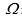,φ) (3)

where kC is the specific cutting resistance (N·mm-1/2), ft is a linear function, taking into account cutting modes,is the angle between wood grains and cutting edge, and φ is the angle between the wood grains and the cutting velocity vector.

A linear model, FC = f(γFρaPDmCφ), was recently published for rotary cutting of two African wood species for three basic cutting cases (modes) (⊥- A, ∥ – B and ∦ – C), employing one wood grain orientation angle φ (Cristóvão et al. 2012). Literature surveys show that the dependence of the main cutting force on all cutting parameters, in a wide range of variation, is non-linear, so the choice of linear model in the cited work does not appear to be appropriate. Furthermore, the use of only one wood grain orientation angle φ to define three basic cutting cases (modes) in one linear model, appears to be a fundamental error. Three orientation angles are necessary for precise definition of cutting cases: φE – between wood grain and the cutting edge, CE; φV – between wood grain and the vector of the cutting velocity, vC, and φC – between the wood grain and the cutting plane (Orlicz 1982; Porankiewicz 2014).

The present study is an attempt to evaluate the relationship between the main (tangential) cutting force, FC, by peripheral milling of oak wood and machining parameters: – cutting depth, cD, and feed per edge, fZ, rake angle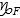, as well as physical properties of wood: – wood density, D, moisture content, mC, and some mechanical properties: – bending strength, RB, modulus of elasticity, E, and hardness, H. The other parameters: – cutting edge round up radius,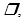cutting speed, vC, – diameter of the cutter, DC, cutter width, WC, number of cutting edges, z, were kept constant. The present study will also make it possible to verify, to a certain degree, the relation K=f(cD, fZ), between relatively cutting resistance K and the cutting depth cD and the feed rate per edge fZ, published in work of Orlicz (1984) for pine wood and applied for oak wood after the use of coefficient of wood species cR=1.55 (1.5 – 1.6).

EXPERIMENTAL

The testing materials included radially cut samples for the determination of physical and mechanical properties of pedunculate oak (Quercus robur).

Physical and mechanical properties were tested in accordance with various standards, i.e., standards for density (SRPS D.A1.044, 1979), for bending strength (SRPS D.A1.046, 1979), for Brinell hardness, perpendicular to the wood grains, in radial direction (EN 1534, 2011) and for modulus of elasticity (SRPS D.A1.046).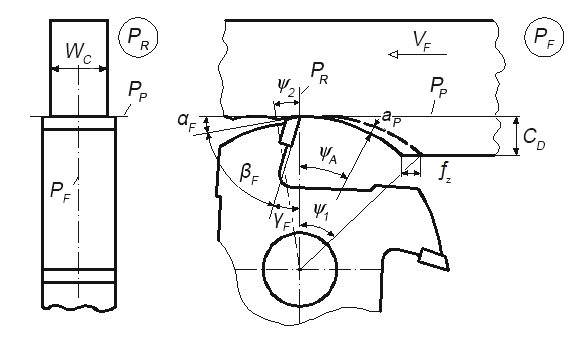Fig. 1. Cutting situation by longitudinal milling with use of the cutter with hole; aP – average thickness of cutting layer;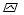A – average meeting angle;1 – maximum meeting angle; P– working plane; P– main plane; P– back plane

Testing during peripheral milling of wood was done on a table-mounted milling machine, MiniMax, equipped in an 3 kW three phase asynchronous electrical motor. Accessory motion was achieved using an Maggi Engineering feeding machine Vario Feed, with speeds ranging between 3 and 24 m∙min-1 and an three phase asynchronous electrical motor nominal power of 0.45 kW. The milling case was up-milling, opened, and peripheral. Three cutters of diameter DC = 125 mm, width CW= 40 mm, equipped with four soldered plates, made of cemented carbide H302 , manufactured by Freud, Italy, were used. The edges had a radius of the round up ρ= 4 um. The roughness of rake and clearance surfaces, after regrinding with grinding wheels, D76, D46 and D7, for respectively rough, finishing final finishing, were respectively of Raa= 0.15 um and Raγ = 0.18 um. The cutters had a clearance angle of aF = 15°, and three different rake angles, γF, namely: 16°, 20°, and 25°. The orientation angle of wood grains towards the cutting edge was φK= 90°. The average wood grain orientation angle toward the cutting speed velocity vector was φV<0.1241; 0.1908 rad>, and that toward the cutting plane was φS<0.1241; 0.1908 rad>.

Testing was done with a constant RPM of the working spindle, i.e., n= 5,860 RPM; at a constant cutting speed, vC= 38.35 m·s-1; at three different feeding speeds, vF= 4, 8, and 16 m·min-1; and at cutting depths of wood cD= 2, 3, and 4.5 mm. The rotational speed of the tool spindle n was measured under load, with the use of a digital, non-contact tachometer, type PCE-DT 65 manufactured by PCE Instruments.

Cutting power was measured indirectly using the power input of the machine driving the electric motor. The measuring device SRD1, connected with a computer, was used for measuring, with sampling frequency of 1kHz. The device uses the Power Expert program package developed in cooperation with the Centre for Wood Processing Machines and Tools and Unolux Company from Belgrade. Figure 2 presents a typical record of cutting power and the manner of its processing.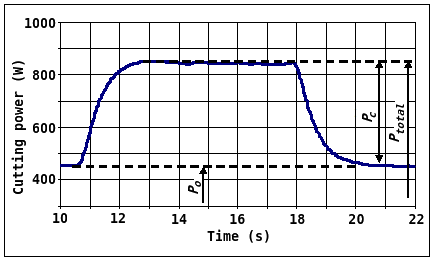Fig. 2. Procedure for processing the results of cutting power measurements during peripheral milling

It can be seen in Fig. 2 that the shape of the record was a rounded trapezium. In the graph, values of total power, Ptotal, power when idle, PO, and power required for cutting, PC, are presented, with the power required for cutting being the difference between the first two values:

PCPtotal – PO (W) (4)

Average values of the measured powers, based on a higher number cuts on the same test sample, were used for calculating mean values of the main cutting resistance by using the following Eq. 5,

FmeanPcmean · vC-1 (N) (5)

where Fmean is the mean value of main cutting force for one revolution of the tool; Pcmean is the average cutting power (W), and vC is the cutting speed (m·s-1).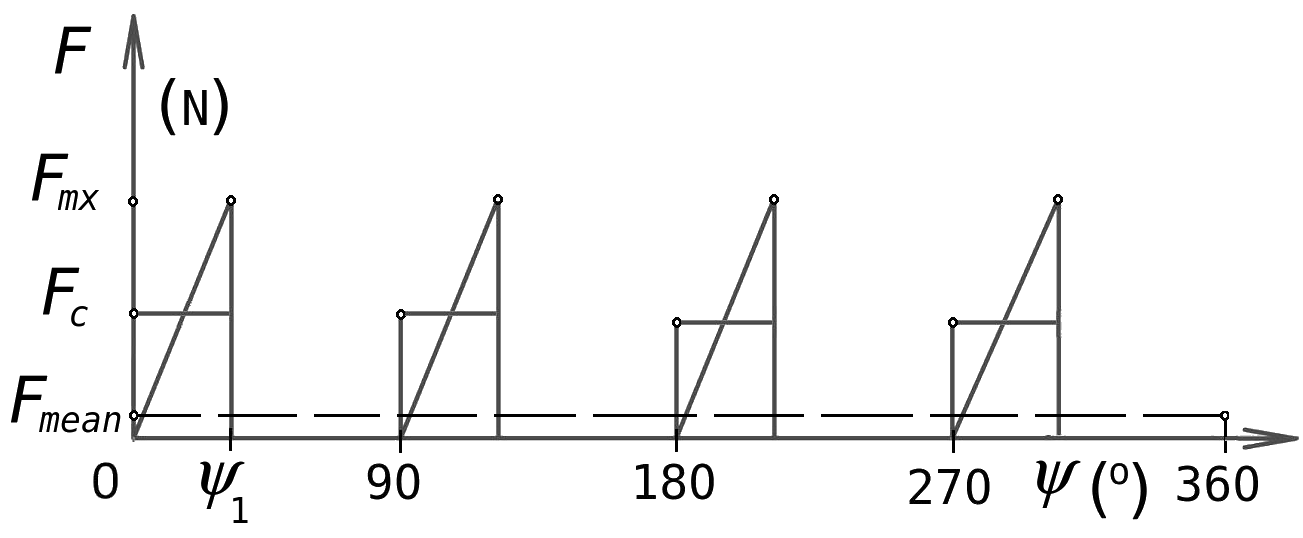The calculated value of the mean force, Fmean, is the mean force for one rotation of the tool, which means that it also includes idle time between the cutting rotations of the four blades (Fig. 3).

Fig. 3. Tangential cutting forces during one revolution of the cutter;– cutting edge rotation angle;1 – maximum edge meeting angle

For obtaining the average main cutting force per one edge, FC, it is necessary to correct the value of mean force, Fmean, as follows,

FC =Fmean ⋅ Ogl ⋅ lr1-1 = Fmean ⋅ 2 ⋅ ⋅  yM )-1 (N) (6)

yM = y1 + y2 (rad) (7)

where Ogl is the circumference of the cutter (m); lr1 is the length of cutting of one edge (m); i is the number of cutting edges; Ris the cutting radius (m); and yM is the rotation angle of the cutting edges in the object (Fig. 1).

For each of the 25 specimens, at least four measurements of physical and mechanical properties were conducted. The measured density, D, values were within the range of 0.613 g·cm-3 to 0.790 g·cm-3, and the calculated mean value was 0.737 g·cm-3. In accordance with the differences in the density, oak hardness, bending strengths, and modulus of elasticity varied. Because the moisture content, mC, of samples varied (from 6.67% to 7.83%, with an average of 7.2%), the values of the wood density computationally were normalized to a humidity of 8%.

As already mentioned, testing was conducted for various combinations of cutting process parameters. In Table 1, the peripheral milling process parameters are given.

Table 1. Peripheral Milling Process Parameters of Oak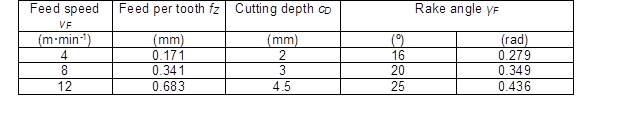Table 2 shows the derived values of a mean thickness of cutting layer (chip thickness), ap, and angle between the cutting velocity vector vCand wood grains,φV.

Table 2. Derived Peripheral Milling Process Parameters of Oak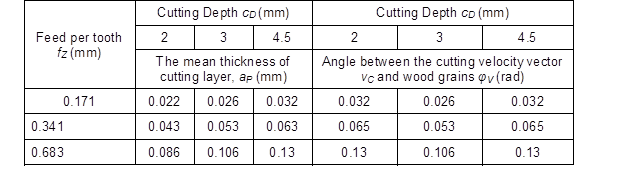The measurements were repeated at least eight times for each combination of processing parameters. Based on the calculated average forces, the relationship FC= f(D8, mC, H, RB, E, fZ, γF, cD) was estimated in preliminary calculations for linear, polynomial, and power types of functions, with and without interactions. The model should fit the experimental matrix by the lowest summation of residuals square SK, by the lowest standard deviation of residuals SR, and by the highest correlation coefficient of predicted, and observed values R. The experimental matrix can be fitted with more simple models, but this will result in decreasing approximation quality, which means that the SK and SR values will increase and R will decrease. It must to be pointed out that any empirical formula can be valid only for ranges of independent variables chosen within the experimental matrix, especially for incomplete experimental matrices and complicated mathematical formula with interactions.

In this case, all predicted values of the dependent variable will have higher expected error. Many years of experience by the authors suggests that efforts to fit such data with overly simple models can be expected to hurt the quality of the approximation, especially in the case of variables with small importance, making such a model nonsensical. The proper influence of low importance variables can be extracted only from an experimental matrix when using a more complicating model. The most adequate formulas appeared to be the non-linear multi variable equations with interactions, Eqs. 8 through 11.

FCP= A+B+C (8)

where

Ae· D8e2 · mCe3 · He4 · RBe5 · Ee6 · fZe7 · γFe8 · cDe9 (9)

B= e10 · f· cD+e11· f· γF+e12 · R· fZ+e13 · R· γF (10)

C=e14 · D· E+e15 · D· fZ+e16 · m· RB+e17 (11)

Estimators were evaluated from an incomplete experimental matrix having 25 data points. During the evaluation process of chosen mathematical models, elimination of unimportant or low important estimators was carried out by use of coefficient of relative importance CRI. The CRI is defined by Eq. 12 and with the assumption CRI > 0.1:

CRI= (Sk+SRI· Sk-1 · 100 (%) (12)

In Eq. 12, the new terms are SK0k, the summation of square of residuals for estimator a= 0. In this equation, ak is the estimator of the number k in the statistical model evaluated.

Figure 4 shows a flow chart of the optimization program used.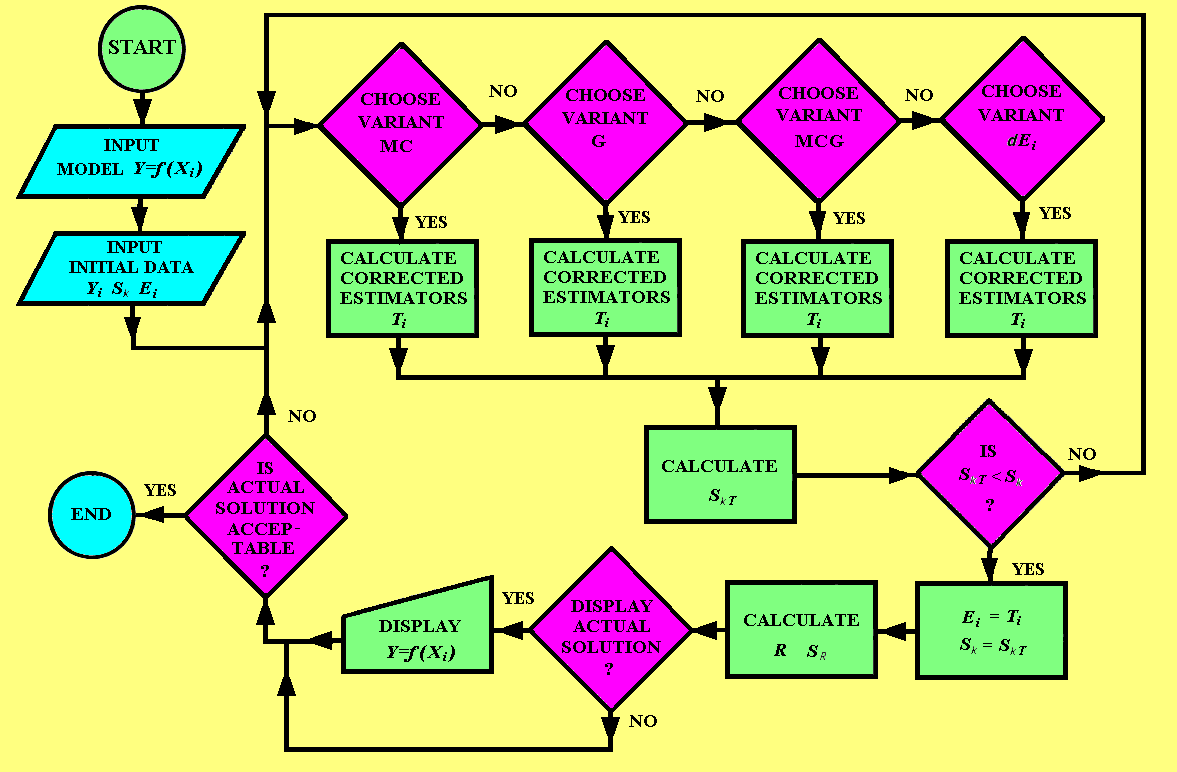Fig. 4. Flow chart of the optimization program; variants: MC- Monte Carlo, G- gradient, MCG- combined MC and G, SK– summation of square of residuals, R– correlation coefficient, SR– standard deviation of residuals

Summation of the residuals squared, SK, standard deviation of residuals, SRcorrelation coefficient, R, and the square of correlation coefficient between predicted and observed values, R2, were used for characterization of approximation quality. The calculation was performed at the Poznań Networking & Supercomputing Centre (PCSS) on an SGI Altix 3700 machine using an optimization program based on a least squares method combined with gradient and Monte Carlo methods (Fig. 4).

RESULTS AND DISCUSSION

The final shape of approximated dependence, Eqs. 8 through 11, using the optimization program shown in Fig. 4, was obtained after 2.84·1010 iterations. The following estimators for were evaluated: e1= 6.89566; e2= 0.071757; e3= 0.44357; e4= 0.01213; e5= 0.4689; e6= 0.2595; e7= 0.056059, e28= -0.22267; e9= 0.013037; e10= 3.072638; e11= -1.7767; e12=-3.40655, e13=0.12584, e14= -0.000040724; e15= 0.41851; e16= -0.86718; e17= -552.38077.

A rounding of estimator values to the 5th decimal place produced an acceptable deterioration of the fit of less than 0.1%. Reducing the number of rounded decimal digits to 4, 3, and 2 would cause unacceptable deterioration of the fit as much as 0.5%, 5668%, 21045%, respectively.

The coefficients of relative importance, CRI, for the estimators have the following values: CRI1=78233531.9, CRI2=11185239.7, CRI3=26717460.2, CRI4=157352.7, CRI5=62689340.4, CRI6= 64980315.8, CRI7=362660, CRI8=69246221.4, CRI9=17637.1, CRI10=729.8, CRI11=10171.1, CRI12= 1276115.8, CRI13=3506721.7, CRI14=4328076.4, CRI15=697231.3, CRI16=21189557.2, CRI17=10851518.3.

For each of the 25 combinations of input data, the predicted tangential cutting forces, Fcp, were calculated using Eqs. 8 through 11. These results are shown in Fig. 5. The approximation quality of the fit, also seen in Fig. 5, can be characterized by the quantifiers SK= 61.86, R= 1.00, R2= 0.99, and SR= 1.76.

Equations 8 through 11, after substituting estimators from e1 to e17and substituting average values of the D8 and the mC, take the following forms,

FCP= A+B+C (13)

where

A= 26.68307 · H0.01213 · RB0.4689 · E0.2595 · fZ0.056059 · γF-0.22267 · cD0.013037 (14)

B= 3.072638 · f· c– 1.7767 · f· γ– 3.40655 · R· f0.12584 · R· F (15)

C=-0.030543 · E + 313.8825 · fZ – 6.27838 · R552.38077 (16)

Figure 5 shows that the maximum deviation from Eqs. 13 through 16 was as high as SR=4.79 N. For minimum and maximum values of the FC, the points lay closer to the regression straight line. These equations provide a strong link between the observed and predicted cutting forces and can be used to analyze the impact of specific inputs on the predicted cutting forces.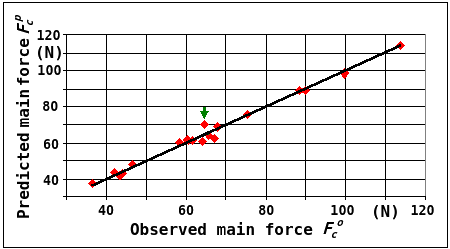Fig. 5. Plot of observed main force FCO against predicted FCPvalues, according to Eqs. 13 through 16

Figure 6 shows a plot of the relationships among the main cutting force, FcP, the feed per tooth, fz, and the rake angle, γF. Figure 6 shows that FCP strongly, non-linearly, in a parabolic decreasing manner depends upon the fZ. An increase in the fZ increased the FCP, more for lower γF. The average change of the FCP with an increase of feed per tooth, fZ, by 0.1 mm is between 7 and 10 N, depending on the size of the rake angle γF. An increase in γF decreases the FCP for the largest feed rate per tooth, fZ. As the fZ fell below ~0.4 mm, a minimum started to appear. For the lowest fZ, the influence of the γFon the FCP was small, with a minimum at γ~21°.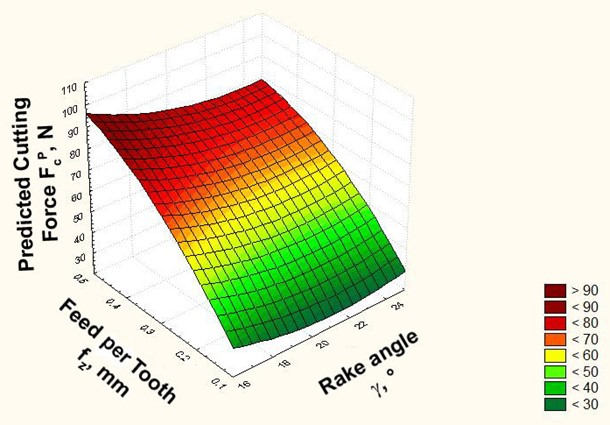Fig. 6. Plot of the relationships among the predicted main force FCP(N), γF (o), and fZ (mm), according to Eqs. 13 through 16; D8 = 0.750 g·cm-3m= 7.24 %; H = 44.16 N·mm-2R=122.47 N·mm-2= 11355.13 N·mm-2c= 3.25 mm

Figure 7 shows a plot of the relationships among the predicted main force, FCP, bending strength, RB (N·mm-2), and wood hardness, (N·mm-2). It can be seen that with an increase in H and RB, the tangential cutting force also grows in a nonlinear, parabolic, decreasing manner. This is consistent with the theory that states that with higher values of bending strength, RB, and/or hardness of wood, H, the resistance to cutting wood grows. This means that the cutting force, FC, will also be greater. From Fig. 7, it can be seen that the influence of bending strength, RB, on the FCP for oak was larger than that of the Brinell hardness, H. When bending strength, RB, increases to 10 N·mm-2, the average change of the cutting force will be as high as 5.3 N. In the case of an increase in hardness, H, by 10 N·mm-2 the cutting force grows by 4.2 N.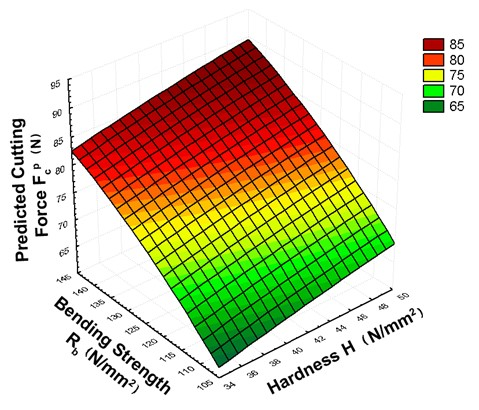Fig. 7. Plot of the relationships among the predicted main force, FCP,H (N·mm-2), and RB (N·mm-2), according to Eqs. 13 through 16; D8=0.75 g·cm-3m=7.24 %; E =11355.13 N·mm-2γF=19.91ofZ=0.427 mm; c= 3.25 mm

Figure 8 shows the relationships among the observed main force, FCP, cutting depth cD (mm), and oak wood modulus of elasticity (N·mm-2). The figure shows a strong, non-linear influence of and the con the main cutting force, FCP. The relationship FCf(cD) combines the influence of increasing thickness of a cutting layer (chip thickness), ap, and to a lesser degree, an influence of increasing grain orientation angle φV. An increase of both independent variables increases the FCP. In the reference range, the overall increase was about 20 N. If the depth of cut increase is 1 mm, the average increase in force, FCP, will be between 7.2 and 7.9 N (lower values are valid for the lower modulus of elasticity E). The influence of the modulus of elasticity E is as follows. When there is an increase in E of 1,000 N·mm-2, the cutting force, FCP, increases by approximately 4 and 16 N in the analyzed range. The analysis of results confirmed that the examined dependency, FCP=f(H, RB, E, fZ, γF, cD), is nonlinear in a range of variation of independent variables.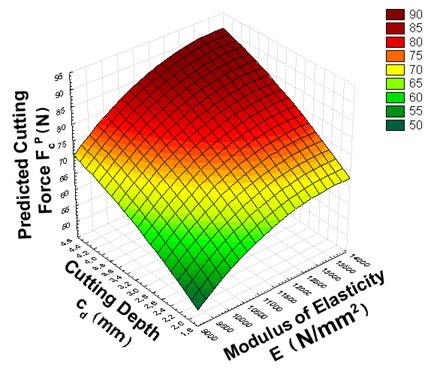Fig. 8. Plot of the relationships among the predicted main force, FCP(N), cD (mm), and E (N·mm-2), according to Eqs. 13 through 16; D8= 0.75 g·cm-3m= 7.24 %; H=46.14 N·mm-2RB= 122.47 N·mm-2γF=19.91o

According to the evaluated formula of relation FC=f(D8, mC, H, RB, E, fZ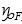, cD) the predicted main cutting force, FCP, decreases with an increase of the wood density D8 in whole range of variation. Similarly, an increase in the moisture content, mC, decreases the FCP, as calculated from this equation, which contradicts the information from the literature (Kivimaa 1950; Franz 1958). For these reasons, the variables D8 and mC were excluded from formula (11) through (16), with assumption D8= 0.75 g·cm-3 and m= 7.24%, and the influence of D8 and mC was not discussed in the work.

The main cutting force, FC (average in one cutting cycle), calculated from formula (11) – (16), evaluated for following average parameters: fZ= 0.427 mm, cD= 3.25 mm,= 19.910D= 0.75 g·cm-3H= 44.16 MPa, RB= 122.47 MPa, and E= 11355 MPa, was of value FC=77.7N. The FC calculated from formulas published in the works Orlicz (1984), Amalitskij and Lûbčenko (1977), Beršadskij (1967) and Orlicz (1982) (fZcD) were respectively: higher 78%, higher 60%, lower 17%, and lower 15%. It has to be mentioned that average values of: D, H, RB and E taken from work of Wagenfür and Scheiber (1974) were respectively: 8% lower, 21 %lower, 28% higher, and 11% higher. It can be seen from this comparison that the values of the FC obtained in the present study lay between values evaluated with use of formulas published in the literature.

CONCLUSIONS

The analysis of results of the calculations performed makes it possible to state the following:

1. An increase in the feed rate per tooth, fZ, increased the main cutting force, FCP, in a parabolic, decreasing manner.
2. For a small rake angle γF of 16°, the established relationship FCP=f(fZ) was strongest.
3. A decrease in the rake angle, γF, for the largest examined feed rate per tooth, fZ, of 0.7 mm increased the main cutting force, FC, in a parabolic, increasing manner. A further decrease in feed rate per tooth, up to f= 0.1 mm, resulted in a decrease in the relationship FCf(fZ), with a minimum of about 21°.
4. An increase of the bending strength, RB, increased the main cutting force, FCP, in a parabolic, decreasing manner.
5. An increase in the Brinell hardness, H, increased the main cutting force, FCP, less than the bending strength RB.
6. An increase in the modulus of elasticity, E, increased the main cutting force, FCP, in a parabolic, decreasing manner.
7. An increase in the cutting depth, cD, increased the main cutting force, FCP, in a parabolic, decreasing manner.

ACKNOWLEDGMENTS

This research was realized as a part of the project “Studying climate change and its influence on the environment: impacts, adaptation and mitigation” (43007) financed by the Ministry of Education and Science of the Republic of Serbia within the framework of integrated and interdisciplinary research for the period 2011-2014.

The authors are grateful for the support of the Poznań Networking & Supercomputing Center (PCSS), Poznań, Poland for a calculation grant.

REFERENCES CITED

Afanasev, P. S. (1961). “Derevoobrabatyvaûŝie stanki” (Woodworking machinery), Lesnaâ promyšlennost’, Moskva.

Amalitskij, V. V., and Lûbčenko, V. I. (1977). “Stanki i instrumenty derevoobrabatyvaûŝh predpriâtij” (Machinery and tools of woodworking factories), Lesnaâ promyšlennost’, Moskva.

Axelsson, B., Lundberg, S., and Grönlund, A. (1993). “Studies of the main cutting force at and near a cutting edge,” Holz als Roh-und Werkstoff 51(1), 43-48. DOI: 10.1007/BF02764590

Beršadskij, A. L. (1967). “Razčet režimov rezaniâ drevesiny” (Resolution of modes of wood machining), Lesnaâ promyšlennost’, Moskva.

Cristóvão, L., Broman, O., Grönlund, A., Ekevad, M., and Sitoe, R. (2012). “Main cutting force models for two species of tropical wood,” Wood Materials Science & Engineering. 7(3), 143-149. DOI: 10.1080/17480272.2012.662996

Deševoy, M. A. (1939). “Mehaničeskaâ tehnologiâ dereva” (Mechanical technology of wood), Lesotehničeskaâ Akademiâ, Leningrad.

Ettelt, B., and Gittel, H. J. (2004). Sägen, Fräsen, Hobeln, Bohren, DRW -Verlag.

Eyma, F., Méausoone, P, and Martin, P. (2004). “Study of the properties of thirteen tropical wood species to improve the prediction of cutting forces in mode B,” Annals of Forest Science 61(1), 55-64. DOI: 10.1051/forest:2003084

Feomentin, G. (2007). “Turning – Cutting forces and power measurements: Comparison of dynamometer force, electrical power and torque current,” COST Action E35, Training School: On- line control and measurements during cutting process, December 5-7, LABOMAP, ENSAM Cluny.

Franz, N. (1958). An Analysis of the Wood-Cutting Process, The Engineering Research Institute, Ann Arbor, MI.

Goglia, V. (1994). “Strojevi i alati za obradu drva I,” University of Zagreb, Faculty of Forestry, Zagreb, 40-78.

Kivimaa, E. (1950). “Cutting Force in Woodworking,” VTT Julkaisu, Helsinki, Finland.

Koch, P. (1964): “Wood Machining Process,” Ronald Press Co., New York.

Krzysik, F. (1975). “Nauka o drewnie” (Wood Science), Państwowe Wydawnictwo Naukowe, Warszawa 1975 (in Polish).

Mandić, M., Mladenović, G., Tanović, Lj., and Danon, G. (2014). “The predictive model for the cutting force in peripheral milling of oak wood,” Proc. of the 12th International Conference on Maintenance and Production Engineering KODIP, June 18-21, Budva, Montenegro, pp. 231-238.

Manžos, F. M. (1974). “Derevorežuŝie stanki,” (Wood Cutting Machinery), Lesnaâ promyšlennost’, Moskva.

McKenzie, W. (1960). Fundamental Analysis of the Wood-Cutting Process, Industry Program of the College of Engineering, Michigan.

McKenzie, W. M., Ko, P., Cvitkovic, R., and Ringler, M. (1999). “Towards a model predicting cutting forces and surface quality in routing layered boards,” Proc. of the 14th IWMS, pp. 489-497.

Naylor, A., and Hackney, P. (2013). “A review of wood machining literature with a special focus on sawing,” BioResources 8(2), 3122-3135. DOI: 10.15376/biores.8.2.3122-3135

Orlicz, T. (1982). “Obróbka drewna narzędziami tnącymi,” (Machining of wood with use of cutting tools), in: Study book SGGW-AR, Warsaw.

Porankiewicz, B. (2014). “Wood machining investigations: Parameters to consider for thorough experimentation,” BioResources9(1), 4-7. DOI: 10.15376/biores.9.1.4-7

Porankiewicz, B., Axelsson, B., Grönlund, A., and Marklund, B. (2011). “Main and normal cutting forces by machining wood of Pinus sylvestris,” BioResources 7(3), 2883-2894. DOI: 10.15376/biores.7.3.2883-2894

Scholz, F., Duss, M., Hasslinger R. J., and Ratnasingam, J. (2009). “Integrated model for the prediction of cutting forces,” Proc. of the 19th IWMS, October 21-23, Nanjing, China, pp. 183 -190.

Time, J. (1870). Soprotivlene Metallov i Dereva Rezaniû, (Cutting resistance of metals and wood), Dermacow Press House, St. Petersburg, Russia.

Tröger, J., and Scholz, F. (2005). “Modelling of cutting forces,” Proc. of the 17th IWMS, Rosenheim, Germany.

SRPS D.A1.044 (1979) according to: ISO 3131:1975 ISO/TC 218, “Wood -Determination of density for physical and mechanical tests,” Serbian standard.

SRPS D.A1.046 (1979) according to: ISO 3133:1975 ISO/TC 218, “Wood – Determination of ultimate strength in static bending,” Serbian standard.

UNE-EN 1534 (2011). “Wood and parquet flooring. Determination of resistance to indentation (Brinell). Test method,” European Committee for Standardization, Brussels, Belgium.

Wagenfür, R., and Scheiber, C. (1974). “Holzatlas,” VEB Fachbuchverlag, Leipzig, (in German).

Woodson, G. E., and Koch, P. (1970). “Tool forces and chip formation in orthogonal cutting of loblolly pine,” Southern Forest Experiment Station, Forest Service, Dept. of Agriculture, New Orleans, LA.

Article submitted: January 14, 2015; Peer review completed: April 19, 2015; Revised version received: May 7, 2015; Accepted: July 9, 2015; Published: July 17, 2015.

DOI: 10.15376/biores.10.3.5489-5502